# Worksheet 3: Definitions and functions

Remember that `rot` can be applied twice to a picture to turn it upside down:

 `rot(rot(man))`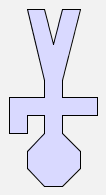`rot(rot(woman))`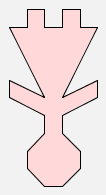It would be convenient to make a new function that could be used when we want two successive rotations to be applied to a picture, as in the examples above. We can do this using an expression with `define`, like this:

`define rot2(p) = rot(rot(p))`

The command `define` makes the expression that follows it on the left hand side of the = sign to stand for the expression on the right of the = sign.

When you type this definition in place of an expression, GeomLab responds with the message

`--- rot2 = <function>`

This shows the `rot2` has been defined as a function: it is not a picture in itself, but it produces a picture when we supply an argument (i.e., the picture that is to be rotated). Having defined the function `rot2`, we can use it in the same way as any other function:

 `rot2(man)`In order to use a defined function in an expression, we just type both the definition and the expression in the input window, like this: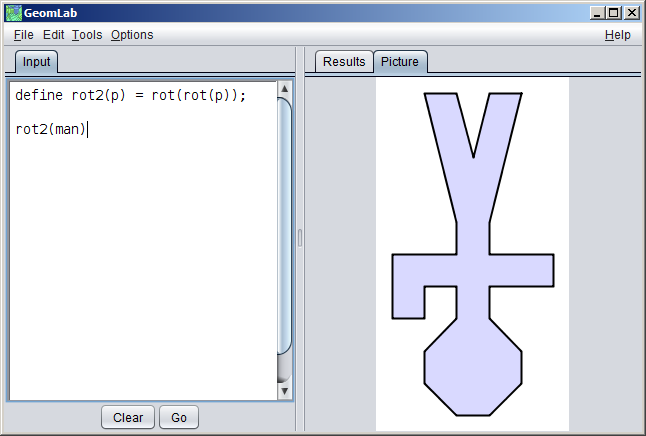Each definition ends with a semicolon, and that helps GeomLab to keep it separate from the following definition or expression.

Replacing `man` by `tree` gives another upside down picture:

 `rot2(tree)`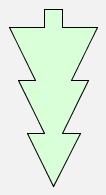Note that the variable `p` used in the expression defining `rot2` is a placeholder. This means that the function `rot2` can be applied to any expression put in place of variable `p`, such as `man` or `woman`.

Let us consider another function definition:

`define f(p) = p \$ (p & p)`

This makes a picture that contains three copies of the argument picture:

 `f(man)`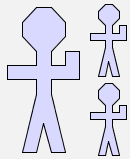`f(woman)`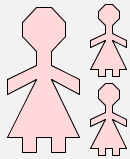What will the image produced by `f(man) \$ tree` look like? Sketch the picture here:

 `f(man) \$ tree`Check your answer in GeomLab: again, you must enter simultaneously both the definition of `f` and the expression that uses it.

Here is the image produced by `f(man \$ tree)`:

 `f(man \$ tree)`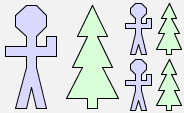This image is different from the one produced by ```f(man) \$ tree```. This is because the brackets in the first example are not around `tree`, so the function `f` is applied only to `man`, whereas the second example has brackets that include `tree`, so the function `f` is applied to the expression `man \$ tree`.

```f(man \$ tree) = (man \$ tree) \$ ((man \$ tree) & (man \$ tree))
f(man) \$ tree = (man \$ (man & man)) \$ tree
```

Now look at the picture `f(f(man))`:

 `f(f(man))`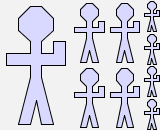Looking at the picture above, there are nine men produced by applying function `f` twice to `man`. What happens if we apply function `f` three times to `man`, as in `f(f(f(man)))`? How many men do you think will be in the picture? Try it!

As described in Worksheet 2, a function takes one or more arguments. For example, the function `f` that we defined earlier takes one argument, which could be `man`, `woman` or any other picture expression.

Let us define a function g that takes two arguments:

`define g(p, q) = (p & q) \$ (q & p)`

Here is what `g(man,woman)` looks like:

 `g(man, woman)`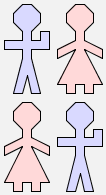What will happen if we type `g(man,g(man,woman))` into GeomLab? Sketch in the space below the image that you think will be produced:

 `g(man, g(man, woman))`